Multiplat

BreizhCTF 2018 - RE (150 pts).

BreizhCTF 2018: Multiplat

Event Challenge Category Points Solves
BreizhCTF 2018 Multiplat Reverse 150 16%

TL;DR

This writeup is about reverse engineering challenge named Multiplat @BreizhCTF. The challenge asks user to enter a base64 encoded file. The program checks character by character the content of a given file. There are many characters I wrote a python script to extract the file. This file is a base64 encoded PE executable which asks a base64 encoded file. I reused my python script to extract the second base64 file which contains the flag.

Static analysis

The associated file to resolve this challenge is here.

The binary asks a base64 encoded file.

``````\$ ./multiplat.elf
Crackme : Validate a base64 file
Usage: ./multiplat.elf <file name>

\$ ./multiplat.elf test.b64
Checking test.b64
File is 13 bytes long.

I'm sorry, Dave, I can't let you do this.
``````

As you can see there is a function check in main which validate the content of given file.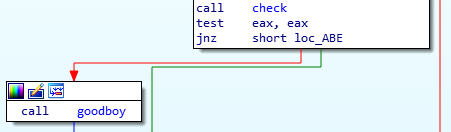The function check is too large to display in IDA because there are too many nodes.If you translate the assembly code above into C, you will obtain the following code

``````index = 0;
if(content[index] ^ 0x1B == 0x4F)
{
[...]
}else
{
}
``````

badboy function displays “I’m sorry, Dave, I can’t let you do this.” and terminate the program. It’s probably not the right path.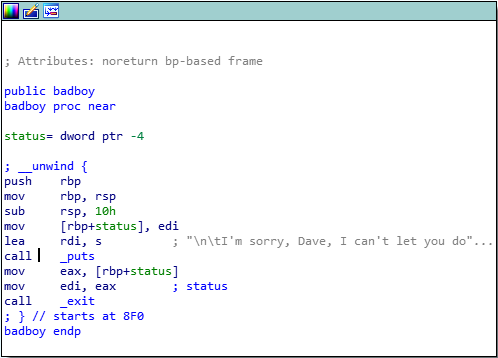So you need to resolve this equation A ^ 0x1B == 0x4F. It will be easy because ^ (XOR) is commutative and associative :

• Message ^ Key = Cipher
• Cipher ^ Key = Message
``````>>> A = 0x1B ^ 0x4F
>>> A
84
>>> print(chr(A))
T
``````

The block of code to check the second character is the same (except the index, and hexadecimal constants).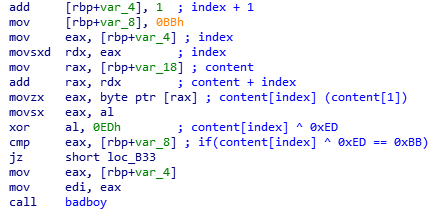Equivalent C code:

``````index = 0;
if(content[index] ^ 0x1B == 0x4F)
{
index+=1;
if(content[index] ^ 0xBB == 0xED)
{
[...]
}
else
{
}
}
else
{
}
``````

But there are too many blocks of code, we need to automate the extraction.

Scripting

Let’s script with IDA Python to extract each characters. First, we need to identify a pattern in block.• The first hexadecimal constant is always stored in var_8.
• The second hexadecimal constant is the operand of the unique xor instruction in the block.

But further in the code, there is another kind of block without XOR instruction.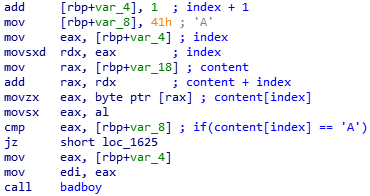Equivalent C code:

``````if(content[index] == 'A')
{
[...]
}
else
{
}
``````

In this case you can directly extract the character.

The script is looking for a mov [rbp+var_8], 0xXX instruction between start_addr and stop_addr

``````import idaapi
import idc

a = ""
b = ""
``````

and a xor reg,0xXX instruction.

``````		# extract second hexadecimal constant
``````

The instruction call badboy indicate the end of block. The script checks if it found two hexadecimal constants. If it’s not the case, that means that there is no xor instruction, the character is hardcoded.

``````		# end of block ?
# 2 hexadecimals constants found ?
if len(b) == len(a) - 1:
# this is a block without xor, so xor the value with null byte
b+="\x00"
# continue until the end of function
``````

Load and run the script with IDA Pro and you will obtain a first base64.The decoded base64 file is a executable for Windows.

``````\$ base64 -d file.b64 > extracted
\$ file extracted
extracted: PE32 executable (console) Intel 80386, for MS Windows
``````

When you open it with IDA, the program is similars to the first binary.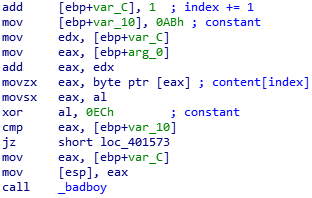Just adapt the script to extract the base64 file.

``````def decipher_string2(start_addr,stop_addr):
a = ""
b = ""
if idc.GetOpnd(addr,0) == "[ebp+var_10]": # [rbp+var_8]

# end of block ?
# check if we found the 2 hexadecimals constants
if len(b) == len(a) - 1:
# if not this is a block without xor, so xor the value with null byte
b+="\x00"

r = ""
for i in range(0,len(a)):
r+=chr(ord(a[i]) ^ ord(b[i]))
print(r)
``````

and the flag is ^^

``````\$ base64 -d file2.b64
Hey this is the final steps.
Go further, don't give up!

Heishiro Mitsurugi is one of the most recognizable characters in the Soul series of fighting games. Mitsurugi made his first appearance in Soul Edge and has returned for all six sequels: Soulcalibur, Soulcalibur II, Soulcalibur III, Soulcalibur IV, Soulcalibur: Broken Destiny and Soulcalibur V. He also appears as a playable character in Soulcalibur Legends and Soulcalibur: Lost Swords, as He Who Lives for Battle.

All I need here is a long text, just because I want you to be able to reverse it. I hope you'll learn some good things. Automatizing things can be really good.

The flag for this challenge is BZHCTF{I_reverse_all_this_and_all_I_got_is_this_flag} without the quotes.

``````